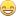## Weighted regression with PROC MCMC

Hello,

I have a data set for which the reliability of certain measurements is known to be superior to others.  I would like to perform quantile regression using PROC MCMC (which I was able to figure out using the SAS documentation), however I would like to weight each datum according to a weight value included in the data set.  Is what I am attempting possible, and if so, how would one go about achieving it?  Please let me know if additional information is required in order to provide an appropriate solution.

Feel free to modify the following hypothetical example (weight can be represented by W) for this purpose:

```proc mcmc data=igg
seed=5263
propcov=congra
ntu=1000
mintune=10
nmc=30000;
begincnst;
p=0.95;
endcnst;
parms (b0-b2) 0;
prior b: ~ general(0);
mu= b0 + b1*age + b2*age2;
u = igg - mu;
ll = log(p)+log(1-p) - 0.5*(abs(u)+(2*p-1)*u);
model igg ~ general(ll);
run;```

*I am using the latest build of SAS v9.4

1 ACCEPTED SOLUTION

Accepted Solutions

## Re: Weighted regression with PROC MCMC

1. The QUANTREG procedure performs quantile regression and supports a WEIGHT statement. Why not use that?

2. For any weighted fit, you need to include the weight variable in the computation of the (log) likelihood function. The "Details" section of the QUANTREG doc shows how to incorporate weights into a quantile regression.  I'm not sure how you derived your log-likelihood function, but maybe modify it to be something like

`u = w*(igg - mu);`

5 REPLIES 5

## Re: Weighted regression with PROC MCMC

1. The QUANTREG procedure performs quantile regression and supports a WEIGHT statement. Why not use that?

2. For any weighted fit, you need to include the weight variable in the computation of the (log) likelihood function. The "Details" section of the QUANTREG doc shows how to incorporate weights into a quantile regression.  I'm not sure how you derived your log-likelihood function, but maybe modify it to be something like

`u = w*(igg - mu);`

## Re: Weighted regression with PROC MCMC

Thanks for your response, Rick.  Regarding your first point, part of my interest in achieving quantile regression via MCMC is to compare its efficacy to QUANTREG for my particular data set.  I also like the idea of knowing the distribution of betas that come with resampling in this manner.  And, somewhat to my surprise, the MCMC procedure solves the same problem more rapidly than QUANTREG (about twice as quickly if I recall correctly), so there is the time factor as well.

Regarding your question about the log-likelihood function, it's taken from an example in the SAS knowledge base ( https://support.sas.com/rnd/app/examples/stat/BayesQuantile/quantile.htm ). It specifies an asymmetric laplace distribution for the dependent variable for the purpose of quantile regression.  My knowledge of different types of distributions is very limited, so I don't claim to know the theoretical basis for this choice of function, however I can say that the betas it generates are very similar to those resultant from the unweighted QUANTREG procedure for the same problem, so I do believe it to be applicable.

If implementing weighting via MCMC is as simple as muliplying the error term by the weight factor as you've described, then that would be splendid.  I will give it a shot and see how the result compares to weighted QUANTREG.

Thanks again.

## Re: Weighted regression with PROC MCMC

Sounds good. I'm certainly not an expert in this area, so experiment and let me know what you discover.  It's good that you'll be able to compare weighted results from the two procedures.

Regarding speed: PROC QUANTREG has four different methods for solving the optimization. Some might be more efficient than others.

## Re: Weighted regression with PROC MCMC

Well Rick, you nailed it.  Your simple modification produced HPD intervals that were improved by a factor of 10 on account of the weighting.  Additionally, the final betas were 99.9% similar to those achieved via weighted QUANTREG.  Both algorithms produced the same R-square, F-statistic, and RMSE, so I think it is safe to say they are converging on the same solution.